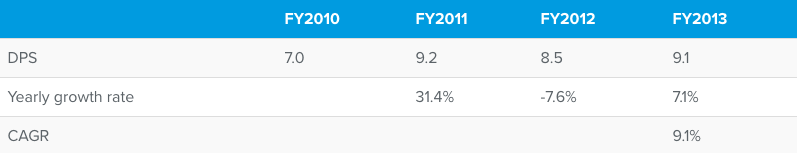Dividend is the portion of company’s profit that is paid out to shareholders. Dividend per share (DPS) refers to the total dividend paid out divided by the common stock of the company. DPS allows investors to earn back a portion of their investment and is an important aspect of stocks returns.

3Y Historical Dividend Growth is calculated as the compounded annual growth rate (CAGR) of DPS over the previous 3 financial years. Compounded annual growth rate is the average annual growth rate of an item over a specified period of time longer than one year. Consider the growth rates of DPS for a few years in the below table:While it is obvious that between FY2010 and FY2013 the DPS of the company increased, the increase and decrease in the interim years does not allow us to understand the approximate yearly growth rate. In such a scenario using compounded annual growth rate is very helpful. CAGR number in the above table has been calculated using the below formula:

((DPS in FY2013 / DPS in FY2010) ^ ( 1/N)) -1, where N is the number of years of growth rate i.e 3 .

Higher the historical DPS growth rate, the better.